NEET  >  Test: Gravitation - 2

# Test: Gravitation - 2

Test Description

## 30 Questions MCQ Test Physics Class 11 | Test: Gravitation - 2

Test: Gravitation - 2 for NEET 2022 is part of Physics Class 11 preparation. The Test: Gravitation - 2 questions and answers have been prepared according to the NEET exam syllabus.The Test: Gravitation - 2 MCQs are made for NEET 2022 Exam. Find important definitions, questions, notes, meanings, examples, exercises, MCQs and online tests for Test: Gravitation - 2 below.
Solutions of Test: Gravitation - 2 questions in English are available as part of our Physics Class 11 for NEET & Test: Gravitation - 2 solutions in Hindi for Physics Class 11 course. Download more important topics, notes, lectures and mock test series for NEET Exam by signing up for free. Attempt Test: Gravitation - 2 | 30 questions in 60 minutes | Mock test for NEET preparation | Free important questions MCQ to study Physics Class 11 for NEET Exam | Download free PDF with solutions
 1 Crore+ students have signed up on EduRev. Have you?
Test: Gravitation - 2 - Question 1

### To complete one revolution around Earth, communication satellites take

Detailed Solution for Test: Gravitation - 2 - Question 1

A communications satellite is in a GEO (geostationary equatorial orbit) with a period of 24 hours. The spin rate ωs about its axis of symmetry is 1 revolution per minute, and the moment of inertia about the spin axis is 550 kg · m2.

Test: Gravitation - 2 - Question 2

### The height above surface of earth where the value of gravitational acceleration is one fourth of that at surface, will be

Test: Gravitation - 2 - Question 3

### The decrease in the value of g on going to a height R/2 above the earth's  surface will be

Detailed Solution for Test: Gravitation - 2 - Question 3

g = GM​/R2
g at height h
gh​ = GM/(R+H)2
h = R/2
gh ​= GM/(R + R/2​)2 ​= GM/((9/4) (R2)) ​= 4/9g
Decrease in the value of g
g − 4/9g = 5/9 g

Test: Gravitation - 2 - Question 4

At what altitude will the acceleration due to gravity be 25% of that at the earth's surface (given radius of earth is R) ?

Detailed Solution for Test: Gravitation - 2 - Question 4

Force on the body placed on Earth's surface is
F = GMm/R2
But, F=mg hence,
mg = GMm​/R2
where, variables have their usual meanings.
gR= GM
Now, force on the body at geo-potential height say h (altitude) where the acceleration due to gravity is 25% of that at the earth's surface i.e.
25//100g ​= g/4​
Hence, we can write
g/4​ = GM/(R+h)2

g/4​ = gR2/(R+h)2

(R+h)= 4R2

Taking roots for both sides we get
R+h = 2R
h = R

Test: Gravitation - 2 - Question 5

If the rotational motion of earth increases, then the weight of the body -

Detailed Solution for Test: Gravitation - 2 - Question 5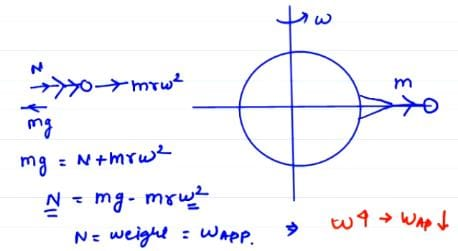Test: Gravitation - 2 - Question 6

On doubling the distance between two masses the gravitational force between them will -

Test: Gravitation - 2 - Question 7

If the acceleration due to gravity inside the earth is to be kept constant, then the relation between the density d and the distance r from the centre of earth will be -

Detailed Solution for Test: Gravitation - 2 - Question 7

If M is the mass of the Earth and m is the mass of a sphere of radius r and you want the force of gravity to be the same at both the surface of the sphere and the surface of the earth then mg = Mg and m = M. This means that the mass of the sphere would have to be the same as the mass of the Earth no matter what r is. This is impossible since a sub-sphere cannot have the same mass as its parent.
For a sphere of radius r to have force g at its surface, d = (De *Re)/r where De is the density of the entire Earth and Re is the radius of the Earth, d is the density of the sphere of radius r. So there is a radius and a density which will produce a gravitational force of g for any d, r >0 but one cannot be a subset of another.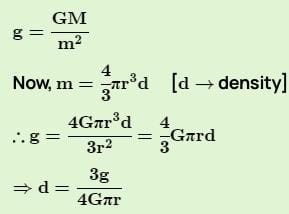= d μ 1/r

Test: Gravitation - 2 - Question 8

Let w be the angular velocity of the earth's rotation about its axis. Assume that the acceleration due to gravity on the earth's surface has the same value at the equator and the poles. An object weighed at the equator gives the same reading as a reading taken at a depth d below earth's surface at a pole (d << R). The value of d is

Detailed Solution for Test: Gravitation - 2 - Question 8

Let,  ge​ be the acceleration due to gravity on surface of earth, g′ be  the acceleration due to gravity at depth d below the surface of earth and is given by
g′=ge​(1−d/R​) ............................(1)
The acceleration due to gravity of an object on latitude(below the surface of earth) is
g′=ge​−ω2Rcosϕ
where, ϕ is the solid angle at the centre of earth sustained by the object.
At the equator, ϕ=0∘
∴cosϕ=1
Hence,
g′=ge​−ω2R ...............(2)
From (1) and (2), we can write
ge​(1−d/R​)=ge​−ω2
⇒dge​​=ω2R
⇒d= ​ω2R2​/ ge

Test: Gravitation - 2 - Question 9

If the radius of the earth be increased by a factor of 5, by what factor its density be changed to keep the value of g the same ?

Detailed Solution for Test: Gravitation - 2 - Question 9

The acceleration due to gravity on surface of earth is
g=GM​/ R2

g=GρV​/R2
where, G is the gravitational constant, M  is mass of earth, R  is radius of earth, ρ is the density and V is the volume of earth.
∴g=Gρ(4/3)​πR3​/ R2

∴g=(4/3)​GρπR
⇒g∝ρR
since, all other quantities are constant.
Hence, if the radius of the earth be increased by a factor of 5, density will decrease by 51​  to keep the value of g the same.

Test: Gravitation - 2 - Question 10

The mass and diameter of a planet are twice those of earth. What will be the period of oscillation of a pendulum on this planet if it is a seconds pendulum on earth ?

Detailed Solution for Test: Gravitation - 2 - Question 10

As Mp​=2Me​ and Dp​=2De
or Rp​=2Re​
Hence, gp​= GMp/(Rp​)2 ​​=G(2Me​)/(2Re​)2 ​= GMe/2Re2​ ​​=ge/2​​
Time period of pendulum on the planet  Tp​=2π√ l/gp​
Tp​=2π√​2l/ge​​=√2​×2π√l/ge​=√2​×Te
Tp​=√2​×2=2√2​s

Test: Gravitation - 2 - Question 11

A particle of mass M is at a distance a from surface of a thin spherical shell of equal mass and having radius a.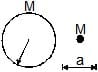Detailed Solution for Test: Gravitation - 2 - Question 11

According to the question, there can be two cases in which the point mass is at a distance a from the surface of the spherical shell.

1. The point mass is at the center of the shell

2. The point mass is outside the shell at a distance 2a from  the center of the shell.

Superposition principle dictates that the potential and field at any point will be the sum of the potentials and fields respectively at that point due to the individual masses.

It can be seen that in both the cases, neither field nor potential is zero inside the shell. This is due to the contribution of the point mass.

Test: Gravitation - 2 - Question 12

If the kinetic energy of a satellite orbiting around the earth is doubled then -

Test: Gravitation - 2 - Question 13

The escape velocity from a planet is v0. The escape velocity from a planet having twice the radius but same density will be -

Test: Gravitation - 2 - Question 14

Two planets A and B have the same material density. If the radius of A is twice that of B, then the ratio of the escape velocity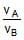is

Detailed Solution for Test: Gravitation - 2 - Question 14

Let the density be d for both the planets. Given that RA​=2RB
Now, mass of A, MA​=4dπRA3/3​=32dπRB3​/3
similarly, MB​=4dπRB3/3
Escape velocity for a planet is given by V=√2GM​​/R
So, VA​=​√2GMA/3RA​​​=√​64GdπRB3/6RB ​​=√32GdπRB​2/3​​

Similarly, VB​=8GdπRB​2/3​​

Taking the ratio, ​VA/VB ​​=32GdπRB​2​/3​×​√3/8GdπRB​2​​=2

Test: Gravitation - 2 - Question 15

A hollow spherical shell is compressed to half its radius. The gravitational potential at the centre

Detailed Solution for Test: Gravitation - 2 - Question 15

Gravitational Potential V = -GM/R for hollow spherical shell at the centre. If we replace R by R/2 then we get V = -2GM/R. Therefore it decreases.

Test: Gravitation - 2 - Question 16

A (nonrotating) star collapses onto itself from an initial radius Ri with its mass remaining unchanged. Which curve in figure best gives the gravitational acceleration ag on the surface of the star as a function of the radius of the star during the collapse ?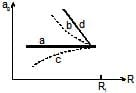Detailed Solution for Test: Gravitation - 2 - Question 16

g∝1​/R2
Curve b represents this variation.

Test: Gravitation - 2 - Question 17

A satellite of the earth is revolving in circular orbit with a uniform velocity V. If the gravitational force suddenly disappears, the statellite will

Detailed Solution for Test: Gravitation - 2 - Question 17

Here the satellite is moving with constant velocity v and it is the gravitational force which is the necessary centripetal force to allow it to move around the earth.
Imagine this as: you are holding a string attached to a stone at other end and you whorl it in the air, now when you suddenly leave the string the stone will move outwards. This is actually tangential to the direction of previously applied force.
When this same concept is applied to the satellite, it will also move away tangentially and with uniform velocity due to zero net force in outer space and no air resistance (as space has vacuum).

Test: Gravitation - 2 - Question 18

A satellite revolves in the geostationary orbit but in a direction east to west. The time interval between its successive passing about a point on the equator is

Detailed Solution for Test: Gravitation - 2 - Question 18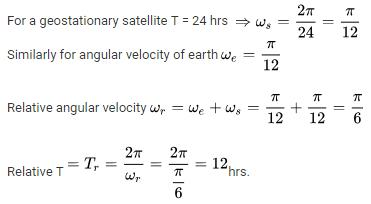Test: Gravitation - 2 - Question 19

Two point masses of mass 4m and m respectively separated by d distance are revolving under mutual force of attraction. Ratio of their kinetic energies will be

Detailed Solution for Test: Gravitation - 2 - Question 19

Let the velocity of m is v and velocity of 4m is u
Therefore, v = 4u and we know that kinetic energy is 1/2mv2 so for m:
KE = ½ x m x v2 = 1
And for 4m:
KE = ½ x 4m x 42 = 4
So, ratio of their kinetic energy is 1:4
Hence A

Test: Gravitation - 2 - Question 20

Select the correct choice(s) :

Detailed Solution for Test: Gravitation - 2 - Question 20

If the TE = 0 that means KE + PE = 0
KE = - PE which is enough for the body to leave the system (here, Earth)

Test: Gravitation - 2 - Question 21

with the orbit radius of a body in circular planetary motion. Find the correct state ment about the

curves A, B and C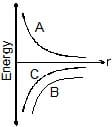Detailed Solution for Test: Gravitation - 2 - Question 21

The body is in circular planetary motion this implies that Potential energy will be negative (attractive)  and the total energy of the system will lie between zero and potential energy (because of circular motion).

Test: Gravitation - 2 - Question 22

Two different masses are dropped from same heights, then just before these strike the ground, the following is same :

Detailed Solution for Test: Gravitation - 2 - Question 22

Both the objects with different masses will attain the same v when striking the ground because acceleration and height is the same.
As, K.E=(1​/2)mv2P.E=−GMm​p/r=mv
Thus K.E, P.E, and p will be different.

Test: Gravitation - 2 - Question 23

When a satellite moves around the earth in a certain orbit, the quantity which remains constant is

Detailed Solution for Test: Gravitation - 2 - Question 23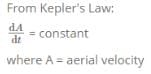Test: Gravitation - 2 - Question 24

A body of mass m rises to height h = R/5 from the earth's surface, where R is earth's radius. If g is acceleration due to gravity at earth's surface, the increase in potential energy is

Detailed Solution for Test: Gravitation - 2 - Question 24

Initial PE = -GMm/R
Final PE = -GMm/R+h, (h=R/5)
Increase in PE = final - initial
= -GMm/R + (R/5) - (-GMm/R)
=GMm(1/R - 5/6R)
=GMm/R * 1/6
= gmR/6 (g= GM/R)
=gm5h/6 (h=R/5)

Test: Gravitation - 2 - Question 25

A planet has mass 1/10 of that of earth, while radius is 1/3 that of earth. If a person can throw a stone on earth surface to a height of 90m, then he will be able to throw the stone on that planet to a height

Detailed Solution for Test: Gravitation - 2 - Question 25

Let mass of earth be M
Acceleration due to gravity = g
Radius of earth = r
Height to which he can throw on earth = H
Acceleration due to gravity at the planet be = g'
Radius of the planet be= r'
Mass of the planet be = M'
Height to which he can throw at that planet = H'
Then g = G(M/r^2)
Therefore g' = G{(1/10)M}/[{(1/3)r}^2]
= (9/10) G(M/r^2)
= (9/10)g
Now, H = {(v)^2-(u)^2}/2(-g)
= {(0)^2-(u)^2}/(-2g)
= {(u)^2}/2g
H' = u^2/[2{(9/10)g}]
= (10/9)u^2/2g
= (10/9)H
= (10/9) * 90
= 100 m

Test: Gravitation - 2 - Question 26

Work done in taking a body of mass m to a height nR above surface of earth will be :

(R = radius of earth)

Detailed Solution for Test: Gravitation - 2 - Question 26

Potential energy at surface of earth will be PE1 = (G.M.m)/R

Now, potential energy at height nR from the surface of earth,

PE2 = (G.M.m)/(R+nR)

Here, change in PE = PE1 – PE2

= (G.M.m)/R – (G.M.m)/(n+1)R

= (G.M.m)/R[1 – 1/(n+1)]

= (G.M.m)/R[(n + 1 – 1)/(n+1)]

= [n/(n+1)](G.M.m)/R ….(i)

Also, g = GM/(R^2) ….(ii)

From (i) and (ii),

Change in PE = (n/(n+1))mgR

Test: Gravitation - 2 - Question 27

The potential energy of a body of mass 3kg on the surface of a planet is 54 joule. The escape velocity will be -

Test: Gravitation - 2 - Question 28

A body of mass m is situated at a distance 4Re above the earth's surface, where Re is the radius of earth. How much minimum energy be given to the body so that it may escape -

Test: Gravitation - 2 - Question 29

A satellite of earth is moving in its orbit with a constant speed v. If the gravity of earth suddenly vanishes, then this satellite will -

Detailed Solution for Test: Gravitation - 2 - Question 29

Obviously, take example that u have one thread in your hand with stone tied at one end and your moving it in vertical circular manner and if u suddenly leave the thread it will go in the direction tangentially to your arm here your hand force is equal to gravitation force but your hand plays centrifugal and gravitation plays centripetal.

Test: Gravitation - 2 - Question 30

The ratio of distances of satellites A and B from the centre of the earth is 1.4 : 1, then the ratio of energies of satellites B and A will be –

Detailed Solution for Test: Gravitation - 2 - Question 30

We know that,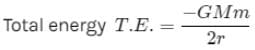Hence the ratio of total energy is in the inverse ratio of the distance ‘r’.

## Physics Class 11

126 videos|449 docs|210 tests
 Use Code STAYHOME200 and get INR 200 additional OFF Use Coupon Code
Information about Test: Gravitation - 2 Page
In this test you can find the Exam questions for Test: Gravitation - 2 solved & explained in the simplest way possible. Besides giving Questions and answers for Test: Gravitation - 2, EduRev gives you an ample number of Online tests for practice

## Physics Class 11

126 videos|449 docs|210 tests

### How to Prepare for NEET

Read our guide to prepare for NEET which is created by Toppers & the best Teachers